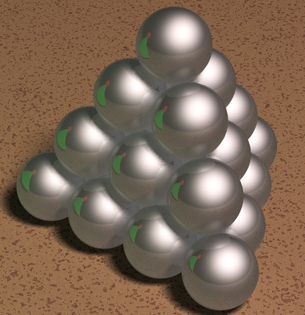Light reflecting off Christmas-tree balls

'Twas the night before Christmas and under the tree
Was a heap of new balls, stacked tight as can be.
The balls so gleaming, they reflect all light rays,
Which bounce in the stack every which way.
When, what to my wondering mind does occur:
A question of interest; I hope you concur!
From each point outside, I wondered if light
Could reach deep inside through gaps so tight?More precisely, let $\cal{B}$ be a finite collection of congruent, perfect mirror balls arranged, say, in a cubic close-packing cannonball stack. Let $H$ be the set of points inside the closed convex hull of $\cal{B}$, $H^+ = \mathbb{R}^3 \setminus H$ the points outside, and $H^- = H \setminus \cal{B}$ the points in the crevasses inside.

Q1. Is it true that every point $a \in H^+$ can illuminate every point $b \in H^-$ in the sense that there is a light ray from $a$ that reaches $b$ after a finite number of reflections?

I believe the answer to Q1 is 'No': If $a$ is sufficiently close to a point of contact between a ball of $\cal{B}$ and $H$, then all rays from $a$ deflect into $H^+$. If this is correct, the question becomes: which pair of points $(a,b)$ can illuminate one another, for a given collection $\cal{B}$? Specifically:

Q2. Is there some finite radius $R$ of a sphere $S$ enclosing a collection $\cal{B}$ such that every point $a$ outside $S$ can illuminate every point $b \in H^-$? More precisely, are there conditions on $\cal{B}$ that ensure such a claim holds?

If the centers of the balls in $\cal{B}$ are collinear, then points in the bounding cylinder do not fully illuminate. If the centers of the balls are coplanar, then points on that plane do not fully illuminate. So some configurations must be excluded. Perhaps a precondition analogous to this might suffice: If the hull $H$ of $\cal{B}$ encloses a sphere of more than twice the common radius of the balls, then ... ? Failing a general result, can it be established for stackings as illustrated above?

The answers (especially Bill Thurston's) in response to the earlier MO question on lightrays bouncing between convex bodies may be relevant. Even speculative 'answers' are welcomed!

Edit (23Dec). Although I remain optimistic that there is a nice theorem lurking here, fedja's observation that points near the boundary of the hull remain dark makes it a challenge to formulate a precise statement of a possible theorem. Something like this:

If $\cal{B}$ is sufficiently "fat," then every point $a$ sufficiently far from $\cal{B}$ illuminates every point $b$ in $H^-$ that is not too close to the boundary of $H$.

Edit (24Dec). There is an associated computational question, interesting even in two dimensions:

Given $a$ and $b$, what is the complexity of deciding if $a$ can illuminate $b$?

Is it even decidable?

• I liked the little poem. :D – J. M. is not a mathematician Dec 22 '10 at 13:58
• What an amazing question and answer!! – Steve D Dec 23 '10 at 8:18
• The question hasn't really been resolved yet because, what the answer really means is that we should state it in some other way. I should say that I feel rather ashamed of all this praise but, once I saw a rhymed question, the temptation to answer in the same style was just too strong :) – fedja Dec 23 '10 at 12:06
• It should be noted, that reflection is only taken in classical way. Because if you take light as in quantum mechanics in consideration, then it is more likely that light will enter and leave. Then light goes in all directions and only points that have light in same phase are illuminated. If due to the configurations, many rays are blocked, then you might get points illuminated, due to the fact there is no out of phase. – Lucas K. Dec 23 '10 at 14:31
• @fedja I do not see how answer is there in your rephrase, can you kindly help me? – Suraj Jain Jul 29 '18 at 6:22

I took a pane of clear glass

and touched two balls at once

I put my light, perhaps, by chance,

above the pane. Alas,

the shining pile on the same side

in its arrangement lay,

and no matter what I tried

(I tried a whole day)

Some darkness (though not too much)

remained around points of touch...

• Beautiful! absolutely beautiful! And the answer is there. – André Henriques Dec 22 '10 at 16:14
• fedja's response is in rhymes so clever / I doubt he'll be topped: Not now, not ever! – Joseph O'Rourke Dec 23 '10 at 1:38
• I'm doubly impressed ... – gowers Dec 24 '10 at 17:45
• Russian. I believe I can speak English more or less fluently by now but, unfortunately, I do it very rarely outside the classroom and the calculus vocabulary is rather limited. So some deterioration is inevitable. – fedja Dec 29 '10 at 13:33
• Dear fedja, you belong to that pantheon of linguistic virtuosi who can handle a foreign language better than 99.99% ( a conservative estimate) of the native speakers. Although I am, alas, completely incompetent to judge the mathematics of your answer, the tenor of the comments, the quality of their authors and the upvotes completely convince me that it is great too. To put it in a nutshell, I consider your post as one of the most extraordinary contributions to MathOverflow since its beginning. – Georges Elencwajg Dec 29 '10 at 21:30

Can't add to the comments (insufficient reputation yet) - I do like the problem and the poetic insight a lot. But here is another less poetic insight.

Q2 can be reversed to look at whether a light at any point $b \in H^-$ will illuminate every point sufficiently distant from it. This seems intuitively more tractable.

• Welcome to Mathoverflow Mark! – gowers Dec 24 '10 at 17:46